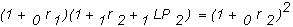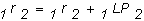office (412) 967-9367
email: ftsweb@gmail.com
toll-free 1 (800) 214-3480

3.10 Theories of the Term Structure of Interest Rates

When you compute forward rates from the ratio of spot rates, as we have described in this chapter, you are applying a purely mathematical relationship, implied from the definition of a spot interest rate. If we assume, however, that the yield curve relates to market expectations about future spot interest rates, we need a theory of term structure behavior to extract this information.

The current US Treasury Yield (this Yield Curve is automatically updated every day) is as follows:

The blue curve is the implied forward rate curve from the current yield curve. To interpret and extract information from this curve we first need to first develkop a theory about the yield curve.

Unbiased Expectations Theory

The unbiased expectations theory assumes that the implied forward rates are an unbiased estimate of the future spot rates. That is, the realized difference between the actual spot interest rate and any previous period’s forward interest rate is, on average, zero. In a world with interest rate uncertainty, as demonstrated by Cox, Ingersoll and Ross  this relationship cannot hold with mathematical equality because of the non linear relationship between price and rates. Practical implications, however, of this observation are minimal.

If the yield curve is upward sloping you can observe that the forward curve lies above the spot curve which under this theory implies that interest rates are expected to increase in the future under unbiased expectations.  By holding the mouse over a dot on the forward curve you can read of the corresponding rate provided in whichever compounding convention you have selected from the dropdown.  The theory underlying how to interpret this number is as follows.

First, recall the distinction between spot and forward rates. An n-period spot interest rate is today’s yield to maturity from a pure discount security (i.e., zero-coupon bond) having a time to maturity equal to n-periods. Equivalently, this spot rate is defined as the geometric average of the one period spot rate and all implied one period forward rates up to period n. For two periods, this is:Under the unbiased expectations theory, this relationship holds in an expected sense. That is, 1 r2 is the spot interest rate that is expected to be realized in one year’s time. These expectations would be supported by expected value-based trading strategies in a world where all investors were assumed to be indifferent to risk.

For example, suppose that the two year spot rate is higher than the geometric average of the one year spot and forward rates. If you cared only about expected returns, you could then make an expected gain by borrowing at the one year spot and forward rates and lending at the two year spot rate.

Applying this principle to the general n-period case, then, all future forward rates are unbiased estimates of future spot rates:Therefore, the n-period spot rate is the geometric average of the one period spot rate and all expected future period spot rates:The unbiased expectations theory of the term structure adds a behavioral interpretation to the mathematical relationships embodied in the term structure of interest rates. That is, the forward rates equal the expected future spot rates, or in other words, forward rates are unbiased estimates of future spot rates.

An implication of the unbiased expectations theory is that, at any one time the debt contracts of different maturities are perfect substitutes for each other. That is, in an expected sense, you are indifferent between investing in long-term government securities and rolling over an investment at the short-term rates.

This implies that all investors are indifferent to interest rate reinvestment risk (i.e., they are risk- neutral). Otherwise, the yield curve embodies risk premium information.

Liquidity Preference Theory

The liquidity preference theory attempts to incorporate risk premium information. It assumes that securities that have different times to maturity are not perfect substitutes for each other, because investors prefer to lend short term and must be offered a "liquidity premium" to be induced to lend long term. As a result, forward rates now provide a biased estimate of future spot rates. The "bias" is the additional premium that must be offered, as a two period example illustrates:whereThis says that the one period forward rate equals the unbiased estimate of the one- period future spot rate plus a liquidity premium for the same period.

It is also assumed that the liquidity premiums increase the farther ahead in time each future period is. As a result, when the unbiased estimate for all future periods is the same, under this theory, the yield curve would appear to be upward sloping a commonly observed shape.

In the above current US Treasury Yield under liquidity premium the forward rates provide biased estimates (i.e., overestimates) of the expected future spot rates where the bias is predicted to get larger with yield to maturity.

Segmented Market Theory

A third theory, the segmented market theory, relies on the fact that different institutional appetites for time to maturity can explain observed shapes of the yield curve. That is, the hedging activities of different sorts of institutions may create different types of clientele for debt securities with different maturity lengths.

For example, pension funds and insurance companies have predominately long-term obligations. The hedging activities of these companies tend to match the maturity of these long-term obligations with the maturity of the fixed-income securities used in the hedge. In Chapter 6, where we cover the bond immunization theorem, you will see why this maturity-matching strategy makes sense. In contrast, many banks have positions that are dominated by shorter term securities, in the form of deposits that have less than a five year life. Maturity matching by these institutions generates a lot of activity at the short end. Finally, at the very short end, the Federal Reserve Bank is the dominant player. Federal reserve activity has a significant impact upon the very short rates. Its activity alters the shape of the yield curve, as evidenced by the events subsequents to the Financial Crisis of 2008.

Interactive Exercise 1:  In the above current US Treasury Yield curve if you look at the short end of the yield curve is this consistent with the Federal Reserve Bank manipulating interest rates?

Interactive Exercise 2:  If you were making a forecast from the forward rate curve of future Federal Reserve Bank behavior relative to interest rates in what direction would you predict they are likely to move and why?

According to the segmented market theory, the demand and supply conditions within each maturity group determine the spot rates for particular maturities.

This theory is not necessarily an arbitrage-free theory, because the spot rates for each level of maturity are not required to depend upon expectations of future spot rates across maturities. In addition, to the extent that there is a clientele for each of the different maturities, the value of the sum of stripped cash flows from a T-note or T-bond may exceed the value of the original unstripped note or bond.